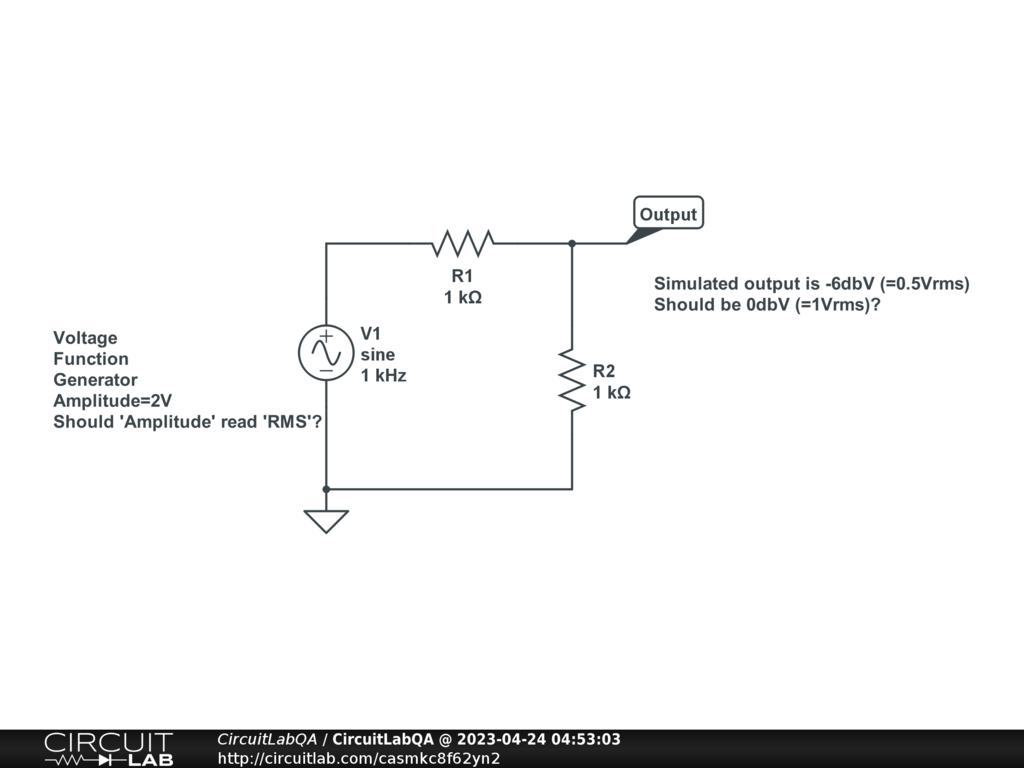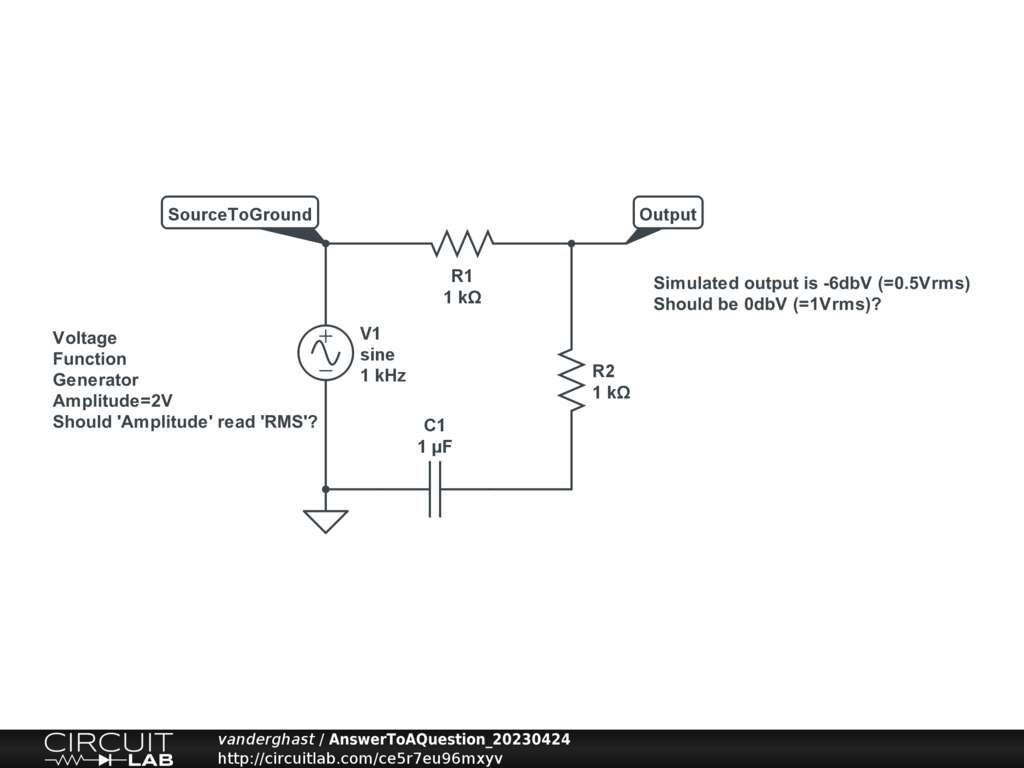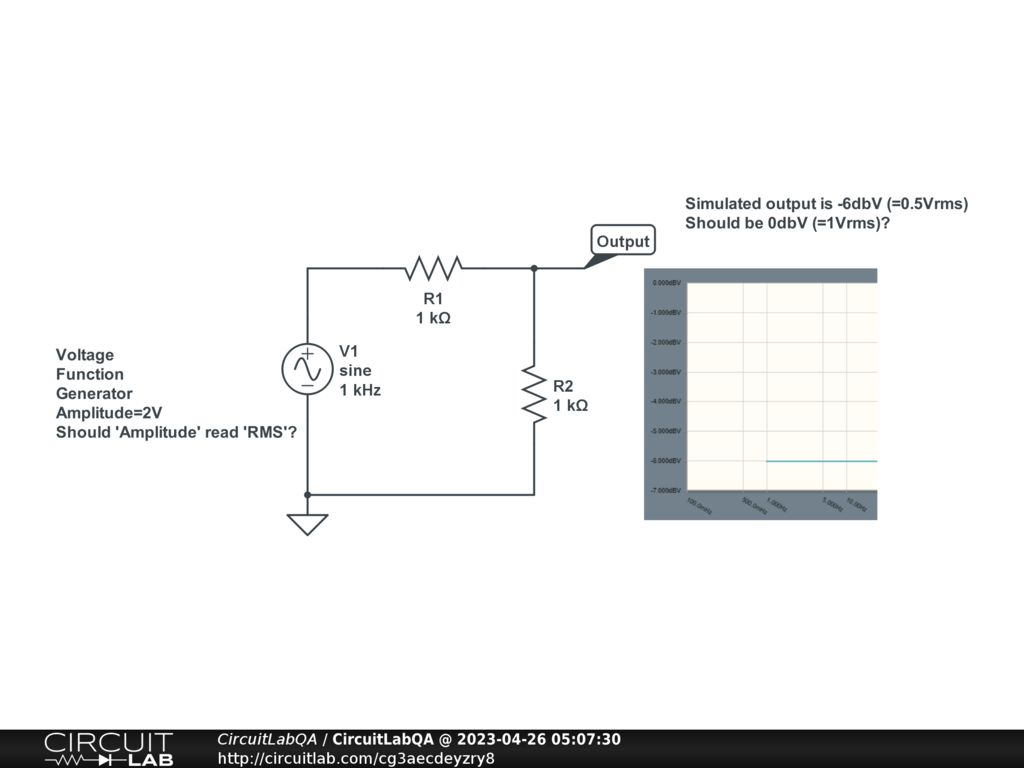## Voltage Function Generator not working?A few questions on the Voltage Function Generator; Changing the 'Amplitude' parameter does not change the level in the Frequency domain simulation. Why is this? Is 'Amplitude' in the parameters (i.e. 1/2 peak to peak voltage) really Vrms? Thanks! by Mark59 April 24, 2023 The amplitude and the frequency are two independent parameters for a source. Changes in one does not change the other. For this simulator (other simulators may have a different definition), the amplitude is half the peak to peak. Vrms is something different. It is the based on squaring the sinus wave (so it is always positive), summing it over a full period, then divided by the length (of the period) and finally, take the square root of this. RMS == Root Mean Square. So, with an amplitude of around 170 volt, you get a Vrms of 120 (typical in North America). Amplitude of 170 is different than the Vrms of 120, and different than Vpp (peak to peak) of 340. Here, I added a capacitor and running a time domain simulation, you should see the sinus wave, and running the frequency simulation, you should see something more useful than for a pure resistive circuit.by vanderghast April 24, 2023Thank you for your response. I may not have been clear enough in my question. I do understand the difference between amplitude and RMS, and what a frequency plot is. The frequency plot has a curve shape (which in this case is flat as there are no complex impedances involved) AND an absolute voltage level which is in this case showing as -6dBV (see image inserted onto diagram). It is this voltage level (not the curve shape) that I am rasing a question about. So, my first question: Is this value of -6dBV correct, given the amplitude on the voltage funtion Generator is set to 2V? And my second question is: Why does't changes in the Voltage Generator Amplitude cause a corresponding change in the dbV level in the frequency plot? Thanks, Mark +1 vote by Mark59 April 26, 2023 Since the circuit is purely resistive and the "output" is at the middle of a voltage divider of 0.5 times the input signal, 20 log(0.5) = -6.0206 At 0 db, the output is of the same magnitude than the input. Since it is a ratio, it is the ratio of the signals which matters, not the amplitude of the input (unless you use some constant voltage drop between the input and the output, such as one implying a diode, as example). by vanderghast April 26, 2023 Yes, I understand dB is a ratio. However, dBV is relative to 1 volt RMS (absolute) i.e. 0dBV = 1 volt; -6dBV = 0.5V; -12dbV = 0.25V, etc. So I was expecting a different absolute dBV level in my voltage divider if the amplitude of the Voltage Function Generator changed...? by Mark59 April 26, 2023 The simulator uses the same definition of dB as in Wikipedia: "The decibel (symbol: dB) is a relative unit of measurement equal to one tenth of a bel (B). It expresses the ratio of two values of a power or root-power quantity on a logarithmic scale." [ I added the bold decorations] A dBV is 20 times, instead of 10 times, since it is about voltage, not power. So it uses the ratio Output/Input. Since the output is directly proportional to the input ( by a factor of 0.5), it does not changes if you change the amplitude of the input, since that will automatically change the amplitude of the output in the same proportion, for the circuit that is of interest here. by vanderghast April 26, 2023 Note that this way of doing is more practical too, since minus 3 dB matches a state where the signal is at 70% of the input, whatever the signal is 1 volt, 5 volt or whatever. And -3dB is what is defined as the bandwidth for an oscilloscope / probes, as example, and that makes that specification independent of the amplitude of the input signal. by vanderghast April 26, 2023 OK, if that is so then maybe the simulator should indicate the vertical axis on the graph as dB and not dBV? WIkipedia specifies dBV as an absolute voltage level (relative to 1 volt - i.e. it is only dependent on the ratio to 1 volt and not any other relative measurement); "dB(VRMS) – voltage relative to 1 volt, regardless of impedance. This is used to measure microphone sensitivity, and also to specify the consumer line-level of −10 dBV, in order to reduce manufacturing costs relative to equipment using a +4 dBu line-level signal" by Mark59 April 26, 2023

CircuitLab's Q&A site is a FREE questions and answers forum for electronics and electrical engineering students, hobbyists, and professionals.

We encourage you to use our built-in schematic & simulation software to add more detail to your questions and answers.

Acceptable Questions:

• Concept or theory questions
• Practical engineering questions
• “Homework” questions
• Software/hardware intersection
• Best practices
• Design choices & component selection
• Troubleshooting

Unacceptable Questions:

• Non-English language content
• Non-question discussion
• Non-electronics questions
• Vendor-specific topics
• Pure software questions
• CircuitLab software support

Please respect that there are both seasoned experts and total newbies here: please be nice, be constructive, and be specific!